Time Estimate

# Override Volume-Based Discounts

## Learning Objectives

After completing this unit, you’ll be able to:

• Configure discount schedules to use amount-based discounts.
• Control when sales reps can change discount schedule details.
• Describe the difference between range and slab types of discount schedules.
• Describe how compound discounts can discount based on quantity as an alternative to discount schedules.

## Percent vs Amount Discount Schedules

Discount schedules are most often configured to reduce a price by a percentage, making them reusable on many products regardless of the product’s price. In other words, a 10% discount works just as well on a \$1.00 key card as it does on a \$2,000.00 copy machine. However, some businesses need to discount by a defined amount instead of a percentage. For example, AW Computing can discount copy paper by \$5.00 per unit for quantities greater than 50. In this scenario, the discount is always \$5.00 even if the price of paper changes from \$85.00 to \$87.00.

To use a fixed amount instead of a percentage for a discount schedule, set the Discount Unit field to Amount.When the discount unit is set to amount, the discount tier editor shows a Discount (\$) field instead of a Discount (%) field.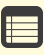#### Note

If your org is configured to use multicurrency, discount schedules that use amount-based discounts require discount tiers for each currency.

## Discount Schedule Override Behavior

Earlier in this module, you created a discount schedule named Bulk Keys that gives 20% off when over 200 key cards are purchased. Automatically applying the 20% discount is convenient for sales reps, but they don’t have any control over the discount schedule values. Thankfully, it’s easy to give sales reps control over the discount tiers while in the Quote Line Editor.In the screenshot above we can see how a sales rep can create a completely new, third tier for the Bulk Keys discount schedule.#### Note

When sales reps save changes in the discount schedule editor, a clone of the original discount schedule is saved and used only for this one quote. The source discount schedule remains untouched. Cloned discount schedules have a lookup field to the original in case sales reps want to revert. They also have lookups to the quote and account records. Lastly, they have a checkbox field named User Defined that is checked, making it easy to identify custom discount schedules.

The field named Override Behavior, found on the Discount Schedule record, controls if sales reps can modify discount tiers. It has has three choices.

• --None--: This makes all values fixed and noneditable by the sales rep.
• All: This lets the sales rep change the upper and lower bounds, as well as the percent or amount for each discount tier. Sales reps have complete control over the discount schedule.
• Current Tier Only: This lets the sales rep change the percent or amount for the Discount Tier based on the current quantity. Although the lower and upper bounds are editable in the user interface, they don’t affect the calculation.

Since editing discount schedules gives sales reps a lot of control over pricing without touching the Additional Discount field, it’s a good idea to create a validation rule or approval process triggered whenever a discount schedule is overridden. Sales reps should also be trained how to add discount tiers properly. If the lower bound of a tier is not equal to the upper bound of the next tier, an error message appears.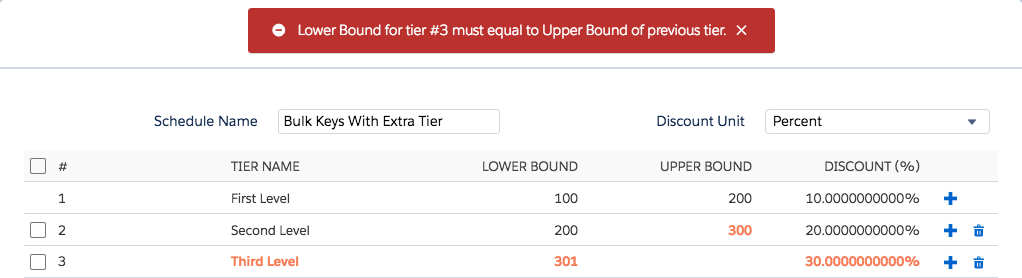## Range vs Slab Discount Schedules

When you created the Bulk Keys discount schedule (as shown in the table below) you found that adding 250 key cards to the quote resulted in a price of \$0.80 per card, for \$200.00 total. In other words, the top tier 20% discount applied to all 250 cards. That’s because the Discount Schedule Type field is set to Range, the default and most common choice.

Tier Name Lower Bound Upper Bound Percent
First Level
100
200
10%
Second Level
200

20%

Setting the Discount Schedule Type to “Slab” results in a very different calculation for the price of the 250 key cards. Instead of applying a single discount percent to all units, the 250 cards are divided into three slabs: 100, 100, and 50. The first slab has no tier defined, so gets no discount. The second slab is for those cards that fall in the First Level tier, so they get 10% off. The last 50 cards are in the Second Level tier, so they get 20% off.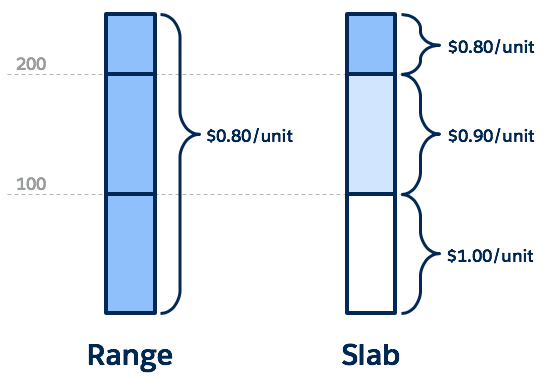The overall price of the key cards when using Slab is calculated like so:

`(100 * \$1.00) + (100 * \$0.90) + (50 * \$0.80)`

Doing the math gives us \$230.00, more than the \$200.00 we got by using the Range type.

Slab discount schedules are perfect for when you need different price-per units for each tier. For example, imagine a “first two free, all others full price” scenario. To set up this discount schedule, you create one tier that’s discounted at 100%, as seen below.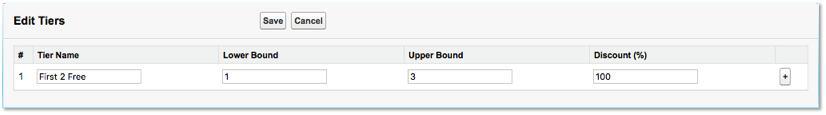When using Slab with this discount tier, if the customer buys five units, the first two are free and the last three aren’t discounted. If we used the Range type, then no units are discounted because there is no tier for five units. This is just one example of many clever ways to leverage Slab discount schedules.#### Note

Slab discount schedules do not work with cross products. To understand why, imagine that we set the Bulk Keys discount schedule to use Slab, and applied it to RFID key cards and RFID key fobs. If you add 30 key cards and 80 key fobs to a quote, you get 110 units overall. With Slab, only 10 units get discounted. But which units get the discount, key cards or key fobs? There’s no way to tell CPQ this information, so cross products with Slab are simply not supported.

## Compound Discount As an Alternative to Discount Schedules

Discount schedules are helpful because you can precisely define quantities that deserve specific discount amounts. However, there’s another way to give volume-based discounts without having to define a discount schedule—a compound discount. This is a way of having a different discount percent for every possible quantity.

Compound discounts are calculated using a mathematical function where we define one parameter. When the function is graphed we see something like this: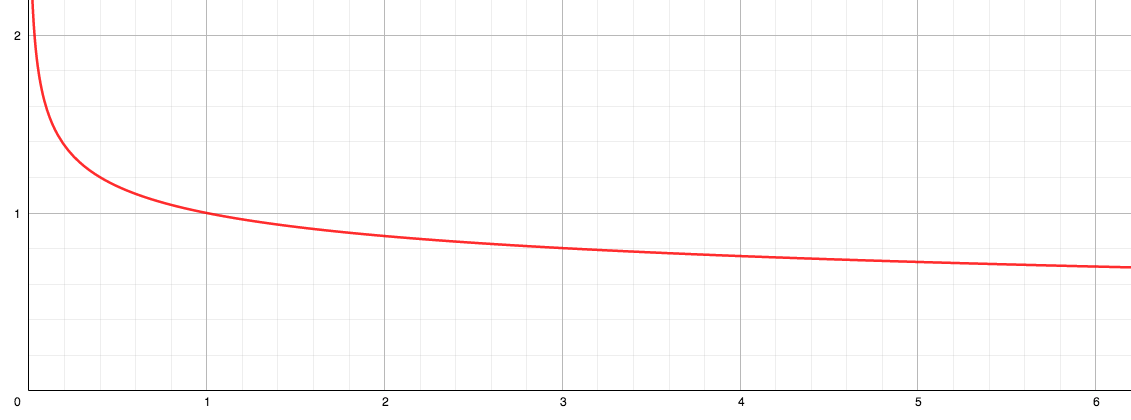Let’s try to make sense out of the graph: The x-axis is quantity and the y-axis is the price multiplier. If we look at 1 unit, the multiplier is exactly 1. In other words, the unit is full price. If the quantity is 2, the multiplier is about 0.87, or roughly a 13% discount per unit. Move over to a quantity of 6 and the multiplier is about 0.7, making for a 30% discount per unit. Every quantity has its own multiplier (which never reaches zero, even at huge quantities).

If you’re curious, the exact function is `1/(Quantity^(Compound Discount/100))`. Salesforce CPQ plugs in the value from the Compound Discount field on the product, does the math, then automatically adjusts the regular price. Different values for compound discount result in different pricing curves; the one in the graph above is for 20%.#### Note

Both compound discount and discount schedules affect regular price, but only the compound discount applies if both are used at the same time.

Good job making it through the math-heavy portion of this module! Now you know just how powerful volume-based discounts can be. In the next unit, things get easier as we see how sales reps can apply discounts manually.

## Resources